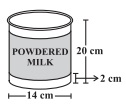# A company packages its milk powder in cylindrical container whose base has a diameter of 14 cm and height 20 cm. Company places a label around the surface of the container (as shown in figure) . If the label is placed 2 cm from top and bottom, what is the area of the label?Given

Diameter of cylindrical container (d) = 14 cm

Radius of cylindrical container (r) = d / 2 = 14 / 2 = 7 cm

Height of cylindrical container (H) = 20 cm

Find out

We have to find out the area of the label

Solution

Height of the label (h) = Height of container – free space

= 20 – 2 (2)

= 16 cm

Area of label = 2πrh

= 2 × (22 / 7) × 7 × 16

= 704 cm²

Hence, the area of the label is 704 cm².